Courses
Courses for Kids
Free study material
Free LIVE classes
More

# The Multi-Digit MultiplicationLIVE
Join Vedantu’s FREE Mastercalss

## Introduction to The Multi-Digit Multiplication

In our earlier classes, we learned about all four maths operations: addition, subtraction, multiplication and division. And from all the operations, our favourites were addition and multiplication because they were simpler than subtraction and division. But today in this article, we will learn about multi-digit multiplication in a very easy and enjoyable way. Doing multiplication is fun if it's done in the right way. So in this article, we will learn about 5 digit multiplication, which we might call hard multiplication. Here we provided multiplication practice problems and 3-digit multiplication worksheets to clear your concepts.

## What is Multiplication?

Multiplication is an important arithmetic operation; it helps to find the total number of items quickly. It's the operation representing the basic idea of repeated addition of the same number. So we can say that it's a method of finding the product of two or more numbers. We use multiplication in our everyday life. Do you want to know how?

So let's say you went to a grocery store to buy 5 apples, which cost 10 rupees. How much money do you need to pay to buy those 5 apples? Do you know the answer? Ok, stop, don't waste your time adding them repeatedly; just multiply 5 by 10, so the answer is 50. So you will need 50 rupees to buy those 5 apples.

Here we understand multiplication and why we need it, we find it interesting, too, so don't worry. We are not going to stop here. We are going to explore multiplication a little bit more. We are going to learn about multi-digit multiplication.

## Symbol of Multiplication

While doing multiplication, there are many symbols which are used to denote the most interesting arithmetic operation, i.e. multiplication. So let's check what symbols are used to denote multiplication.

Cross sign “X”, dot sign “.”, and the star sign “*”. And remember, if there is no symbol after the bracket, there is also multiplication between the numbers. Confused? Don't worry. Let's check it with an example 5(7)= 35.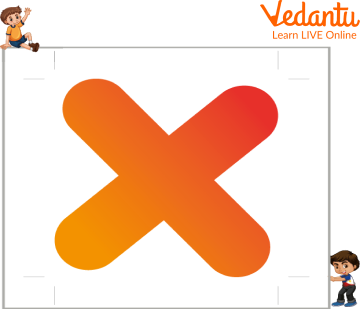Symbol of multiplication

## Formula for Multiplication

The multiplication formula is very simple. Let's see what it is, Multiplicand x Multiplier = Product. Where multiplicand refers to the first number, the total number of objects in each group. Then Multiplier is the second number which is the number of equal groups.

And the last is the product which is the final result that we get after multiplying the multiplicand and the multiplier.

Example: If a multiplier is 6 and multiplicand is 5, the product is 30.

12 x 10 = 120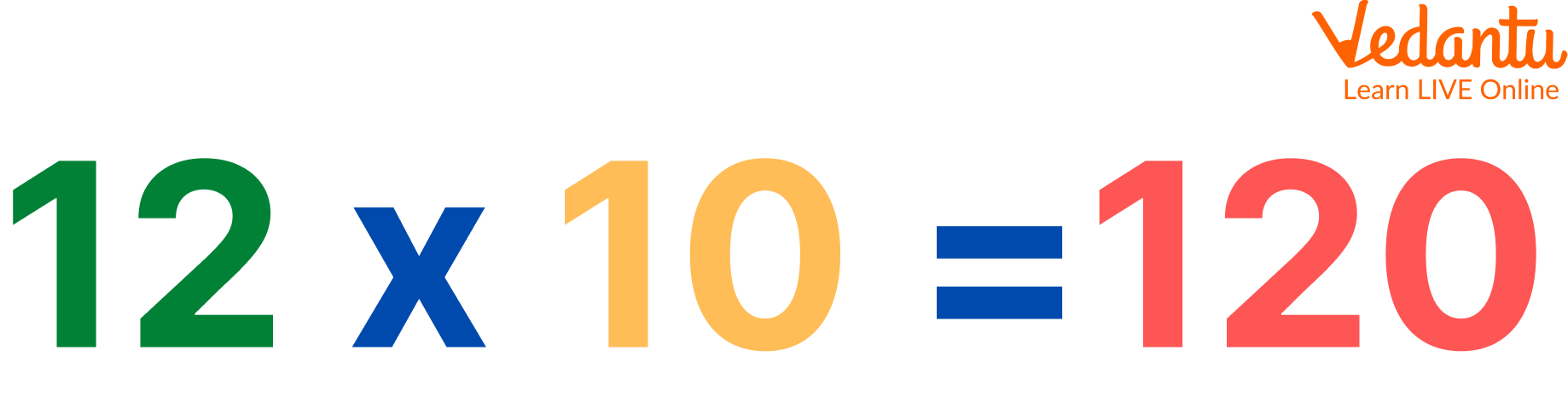Multiplication

## Rules for Multiplication

• If any number is multiplied by 0, the product will be 0.

Example: $5 \times 0 = 0$

• The multiplication of two integers is an integer.

Example: $5 \times 5 = 25$

• The order of the number does not matter when they are multiplied together.

Example: $25 \times 5 = 125$ or $5 \times 25 = 125$

• If any number multiplied by 1 is equal to the original number.

Example: $5 \times 1 = 5$

## Solved Example

Let's learn about multiplication by looking at solved examples based on 5-digit multiplication and 3-digit multiplication:

Q 1. Multiply 123 x 3

Ans: Step-1. Write the number one below the other as per the places of their digits.Multiplication

Step-2. Multiply the ones digit of the top number by the ones digit of the bottom number.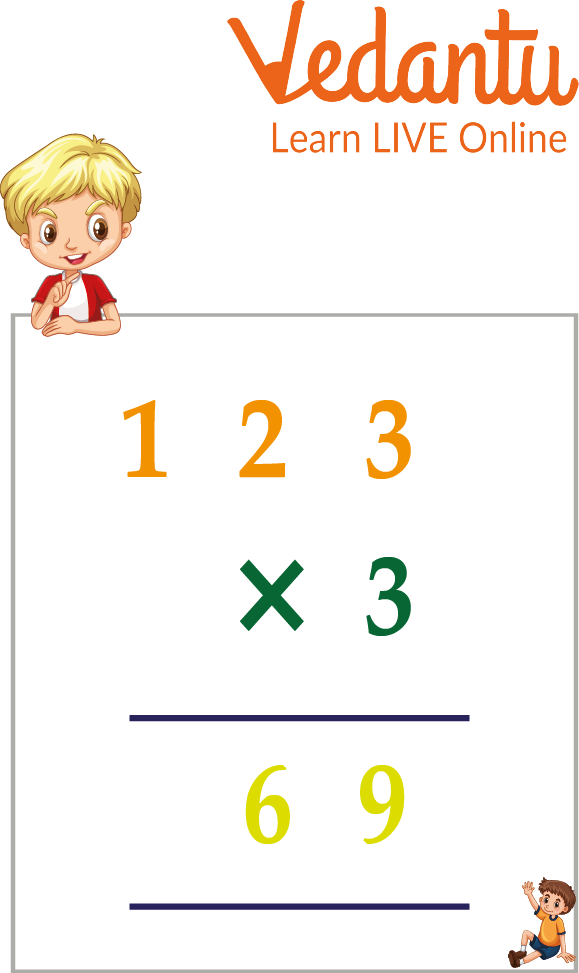Multiplication

Step-3. Multiply the tens digits of the top by the ones digit of the bottom number and you will get your answer.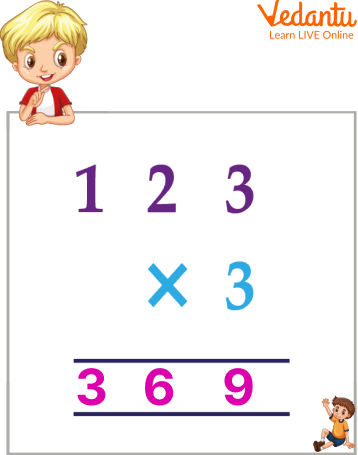Multiplication

Q 2. Multiply 36953 x 3

Ans: Step-1. Write the number one below the other as per the places of the digit.

Step-2. Now by multiplying the tens place, if there is a carry of number, shift it to another number at the top of the hundreds place.

Step-3. By multiplying in this way, you will get your answer. i.e. 110859.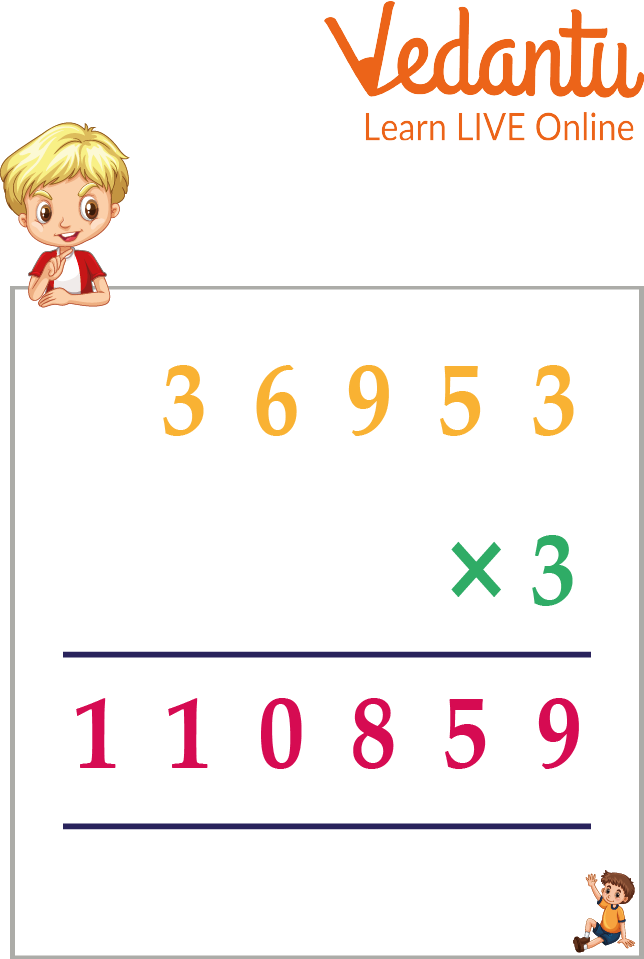5-digit multiplication

## Multiplication Practice Problems

Here are some practice problems based on hard multiplication:

Q 1. 343 x 213

Ans. 73,059

Q 2. 369 x 15

Ans. 5535

Q 3. 513 x 33

Ans. 16,929

## 3 Digit Multiplication Worksheets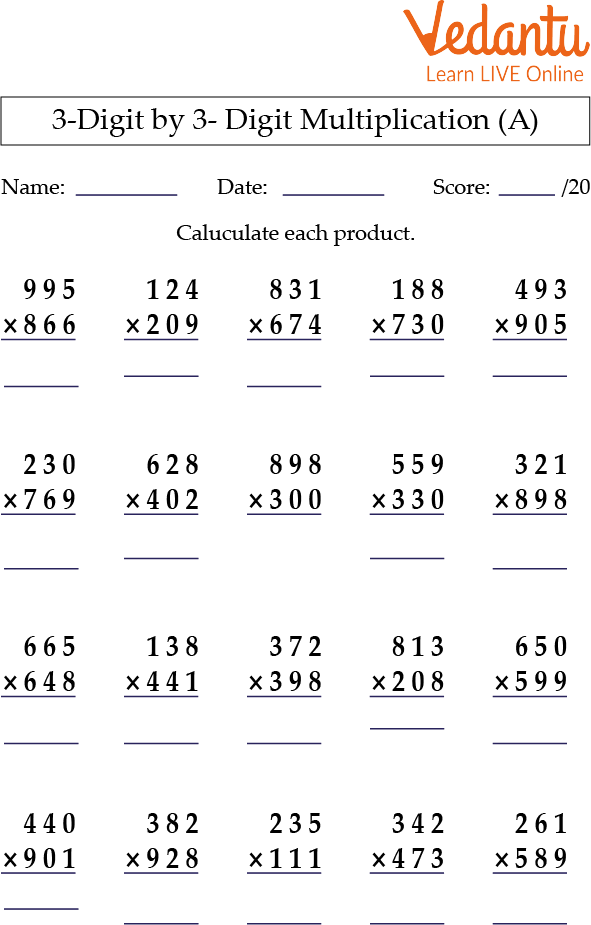3-Digit Multiplication Worksheets

## Summary

So in this article, we learned about many wonderful things related to multiplication. We learned about multiplication, like what multiplication is and what are the symbols of multiplication. We learned the formula of multiplication. We also discussed the rules for multiplication to make hard multiplication easy.

We just did not end there. We also did some multiplication practice problems as we know that maths is not learned by reading. It's learned by solving by doing questions.

That's why we have provided a 3-digit multiplication worksheet to clear all your doubts.

Last updated date: 22nd Sep 2023
Total views: 80.4k
Views today: 1.80k

## FAQs on The Multi-Digit Multiplication

1. What are various ways to multiply?

Addition method, long multiplication, the grid method, and the drawing line method.

2. What is a synonym for multiply?

Augment, enlarge, and increase are the synonyms of multiply.

3. Is it important to learn tables to solve multiplication problems?

Yes, it's important to learn tables to solve multiplication problems.